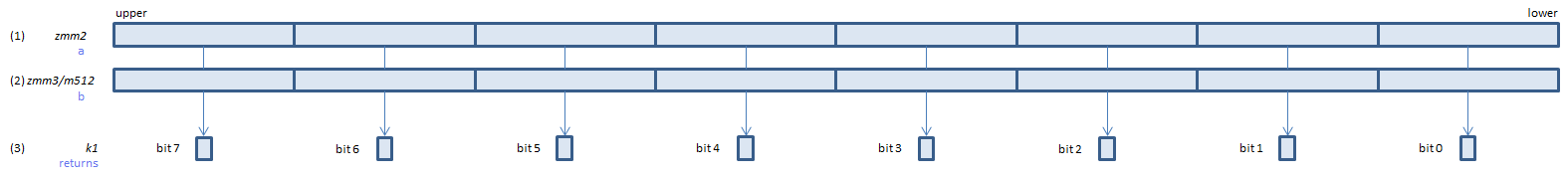﻿ vptestmq

## VPTESTMQ - Packed TEST Mask Qword

VPTESTMQ k1{k2}, xmm2, xmm3/m128/m64bcst    (V5+VLFor each QWORD, calculate bitwise (1) & (2), if any bit of the result is 1, set 1, else set 0, to the corresponding bit of (3).
If k2 bit is 0, the calculation is not done and the corresponding bit of (3) is set to zero. Upper bits of (3) are zero cleared.
VPTESTMQ k1{k2}, ymm2, ymm3/m256/m64bcst    (V5+VL